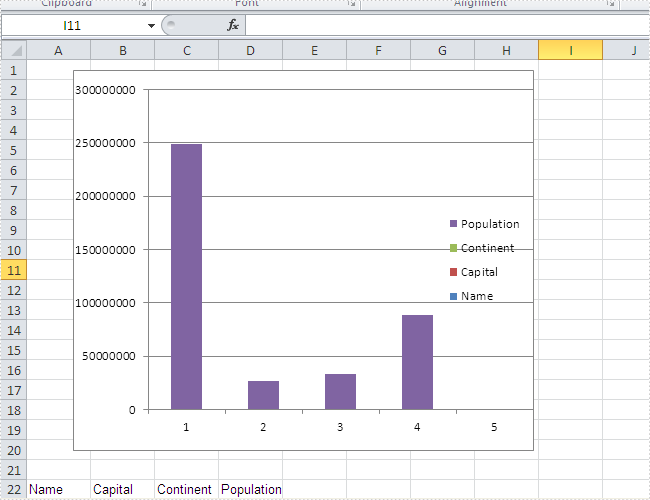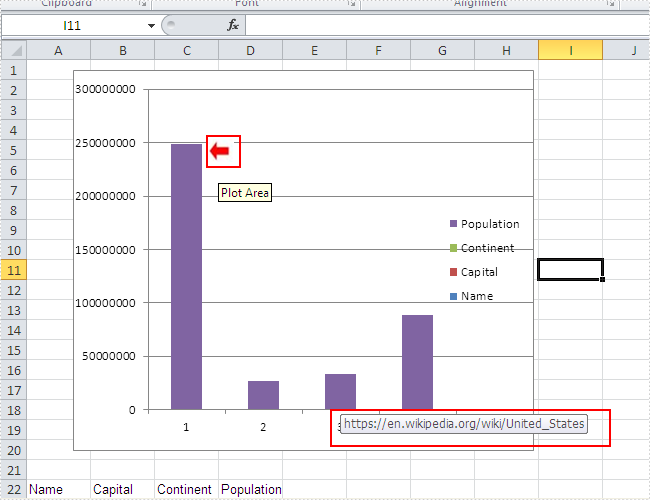# How to add a picture to the chart and then assign a hyperlink to the picture

One of our users requests a demo of Spire.XLS for how to add a picture to the chart at a specified location and then assign a hyperlink to the picture in C#. To fulfil it, we need to prepare the two things. Firstly, we need to know how to create Excel Charts in C# with the help of Spire.XLS. Secondly, we should have an image that we use to insert to the chart. We will use Excel Column chart for example.

Here comes to the steps. Firstly, please review the sample excel chart that we will add image later.Step 1: Create a new document and load from file.

```Workbook workbook = new Workbook();
workbook.LoadFromFile("sample.xlsx");
```

Step 2: Get the first worksheet and the first chart in it.

```Worksheet workSheet = workbook.Worksheets;
Chart chart = workSheet.Charts;
```

Step 3: Add the desired image into the chart and set the image's position and size.

```IPictureShape ps = chart.Shapes.AddPicture("1.png");
ps.Top = 180;
ps.Left = 280;
ps.Width = 60;
ps.Height = 80;
```

Step 4: Assign a hyperlink to the image.

```(ps as XlsBitmapShape).SetHyperLink("https://en.wikipedia.org/wiki/United_States", true);
```

Step 5: Save the document to file.

```workbook.SaveToFile("result.xlsx", ExcelVersion.Version2010);
```

Effective screenshot of adding an image to the chart and assign a hyperlink to the image:Full codes:

```using Spire.Xls;
using Spire.Xls.Core;
using Spire.Xls.Core.Spreadsheet.Shapes;
namespace AddPicturetoChart
{
class Program
{

static void Main(string[] args)
{
Workbook workbook = new Workbook();
workbook.LoadFromFile("sample.xlsx");

Worksheet workSheet = workbook.Worksheets;
Chart chart = workSheet.Charts;

IPictureShape ps = chart.Shapes.AddPicture("1.png");
ps.Top = 180;
ps.Left = 280;
ps.Width = 60;
ps.Height = 80;
(ps as XlsBitmapShape).SetHyperLink("https://en.wikipedia.org/wiki/United_States", true);

workbook.SaveToFile("result.xlsx", ExcelVersion.Version2010);
}
}
}
```